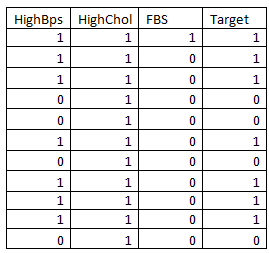top of pageSearch

# Understanding the Gini Index in Decision Tree with an ExampleA decision tree is a specific type of flow chart used to visualize the decision-making process by mapping out the different courses of action, as well as their potential outcomes. Decision trees are vital in the field of Machine Learning as they are used in the process of predictive modeling.

In Machine Learning, prediction methods are commonly referred to as Supervised Learning. Supervised methods are an attempt to discover a relationship between input attributes(or independent variables) and a target attribute (or dependent variable). The relationship that is discovered is represented in a structure referred to as a Model.

The model describes and explains phenomena that are hidden in the dataset and which can be used for Predicting the value of the target attribute whenever the values of the input attribute are known.

The supervised models are of two types:

1. Classification Models(classifiers)

2.Regression Models

Classifiers: They deal with when the data is in the discrete or categorical form. For example, classifiers can be used to classify an object or an instance such quality of product such as good or bad(damaged). Another example is a hot day or a cold day.

Regression models: They deal with data that are continuous. For instance, a regressor(independent variable) can predict the demand for a certain product given its characteristics. Example sales of the product for a company can be predicted based on advertising done on the product. Another example is what is the temperature today means the exact value.

A decision tree is self-explanatory as they follow the hierarchical structure which makes it easy to understand. The entire goal of the Decision tree is to build a model that can predict the value of a target variable based on the decision rules that can be inferred from the features. One thing to note is that the deeper the tree is, the more complex the rules are.

How to identify the attribute?

In Decision Tree, the major challenge is to identify the attribute of the root node in each level. This process is known as attribute selection. We have two popular attribute selection measures:

The Gini impurity measure is one of the methods used in decision tree algorithms to decide the optimal split from a root node and subsequent splits.

Gini index is also known as Gini impurity.

What is the Gini Index?

Gini index calculates the amount of probability of a specific feature that is classified incorrectly when selected randomly.

If all the elements are linked with a single class then it is called pure.

It ranges from 0-1

0 = all elements

1 = Randomly distributed

0.5 = equally distributed

It means an attribute with a lower Gini index should be preferred.Equation of Gini index:where pi is the probability of an object being classified to a specific class.

Gini index example

Now we will understand the Gini Index using the following table :

It consists of 14 rows and 4 columns. The table depicts on which factor heart disease occurs in person(target) dependent variable depending on HighBP, Highcholestrol, FBS(fasting blood sugar).

Note: The original values are converted into 1 and 0 which depict numeric classificationDecision tree for the above table:

when a decision tree is drawn for the above table it will as followsDecision tree

How the tree is spilted?

1. Target is the decision node.

2. It is subdivided into the parent node (Highbps, High cholesterol, FBS)

3. Parent node is divided into child node basing on the value of how many 1 or 0 in parent node such as EX: HBPS1 &HBPS0

4. These are again divided into leaf node based on target=1 & target=0

(leaf node is end node, it cannot be further divided)

Now let calculate the Gini index for Highbps, Highcholestrol, FBS and will find the one which factor decision is made.

The factor which gives the least Gini index is the winner, i.e,.based on that the decision tree is built.

Now Finding Gini index for individual columns

1. Gini index for High Bps:

Decision tree for High BPSProbability for parent node:

P0= 10/14

P1=4/14

Now we calculate for child node :

i) For BPS=1,

for bps =1 this is the tableIf (Bps=1 and target =1)=8/10

if(Bps=1 and target=0)=2/10

Gini index PBPS1=1-{(PBPS1)2+(PBPS0)2

= 1-{{8/10)2+(2/10)2}

=0.32

2) if BPS=0,If (BPS=0 and target=0)=4/4=1

If (BPS=0 and target=1)=0

Gini index PBPS0=1-{(1)-(0)}

= 1-1

=0

Weighted Gini index

w.g =P0*GBPS0+ P1*GBPS1

= 4/14*0 + 10/14*0.32

= 0.229

2. Gini index for High Cholestrol:Decision Tree for High Cholestrol

Probability of parent node

P1=11/14

P0=3/13

i) For HChol.=1If (Hchol.=1 and target=1)=7/11

If (HChol.=1 and target=0)=4/11

Gini index = 1-[(7/11)2+(4/11)2]

= 0.46

ii) If HChol.=0If (Hchol.=0 and target=1)=1/3

If (HChol.=0 and target=0)=2/3

Gini index= 1-[(1/3)2+(2/3)2]

= 0.55

Weighted Gini index = P0*GHChol.0+P1*GHChol.1

= 3/14*055+11/14*0.46

= 0.47

3. Gini index for FBPS:Decision tree for FBPS

Probability of parent node

P1=2/14

P0=12/14

i) for FBPS=1If (FBps=1 and target =1)=2/2

if(FBps=1 and target=0)=0

Gini index PFBPS1=1-{(PFBPS1)2+(PFBPS0)2

= 1- [(1)2+0]

=1-1=0

ii) for FBPS=0,If (FBps=0 and target =1)=6/12=0.5

if(FBps=0 and target=0)=6/12=0.5

Gini index PFBPS0=1-{(PFBPS1)2+(PFBPS0)2]

= 1-[(0.5)2+(0.5)2]

= 0.5

Weighted Gini index = P0*GFBPS0+ P1*GFBPS1

= 6/7*0.5+1/7*0

=0.42

Comparing Gini Index:As HighBPS is less it is the winner

Conclusion: HighBPS is used as the root node for constructing of Decision Tree and the further tree is built.